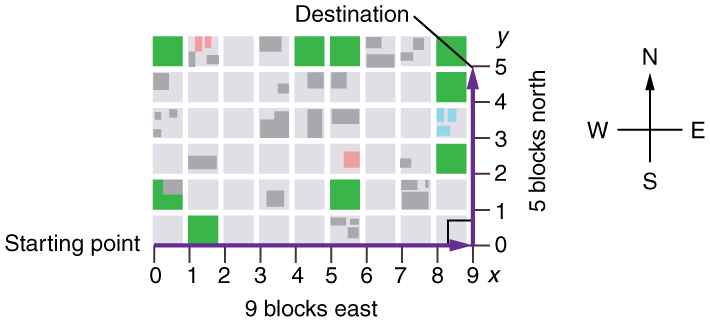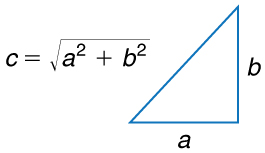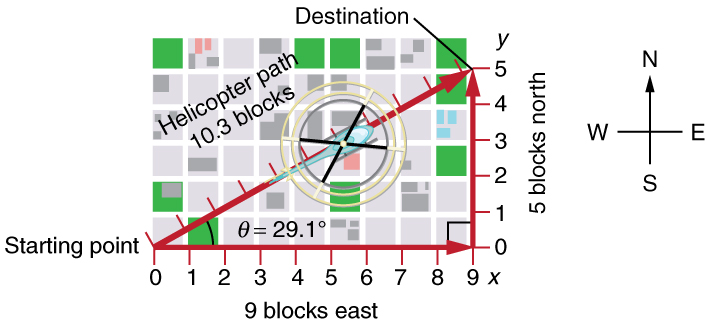# 3.1 Kinematics in two dimensions: an introduction

 Page 1 / 3
• Observe that motion in two dimensions consists of horizontal and vertical components.
• Understand the independence of horizontal and vertical vectors in two-dimensional motion.Walkers and drivers in a city like New York are rarely able to travel in straight lines to reach their destinations. Instead, they must follow roads and sidewalks, making two-dimensional, zigzagged paths. (credit: Margaret W. Carruthers)

## Two-dimensional motion: walking in a city

Suppose you want to walk from one point to another in a city with uniform square blocks, as pictured in [link] .A pedestrian walks a two-dimensional path between two points in a city. In this scene, all blocks are square and are the same size.

The straight-line path that a helicopter might fly is blocked to you as a pedestrian, and so you are forced to take a two-dimensional path, such as the one shown. You walk 14 blocks in all, 9 east followed by 5 north. What is the straight-line distance?

An old adage states that the shortest distance between two points is a straight line. The two legs of the trip and the straight-line path form a right triangle, and so the Pythagorean theorem, , can be used to find the straight-line distance.The Pythagorean theorem relates the length of the legs of a right triangle, labeled a size 12{a} {} and b size 12{b} {} , with the hypotenuse, labeled c size 12{c} {} . The relationship is given by: a 2 +  b 2 =  c 2 size 12{a rSup { size 8{2} }  "+ "b rSup { size 8{2} }  "= "c rSup { size 8{2} } } {} . This can be rewritten, solving for c size 12{A} {} : c  =  a 2 +  b 2 size 12{c" = " sqrt {a rSup { size 8{2} }  "+ "b rSup { size 8{2} } } } {} .

The hypotenuse of the triangle is the straight-line path, and so in this case its length in units of city blocks is , considerably shorter than the 14 blocks you walked. (Note that we are using three significant figures in the answer. Although it appears that “9” and “5” have only one significant digit, they are discrete numbers. In this case “9 blocks” is the same as “9.0 or 9.00 blocks.” We have decided to use three significant figures in the answer in order to show the result more precisely.)The straight-line path followed by a helicopter between the two points is shorter than the 14 blocks walked by the pedestrian. All blocks are square and the same size.

The fact that the straight-line distance (10.3 blocks) in [link] is less than the total distance walked (14 blocks) is one example of a general characteristic of vectors. (Recall that vectors are quantities that have both magnitude and direction.)

As for one-dimensional kinematics, we use arrows to represent vectors. The length of the arrow is proportional to the vector’s magnitude. The arrow’s length is indicated by hash marks in [link] and [link] . The arrow points in the same direction as the vector. For two-dimensional motion, the path of an object can be represented with three vectors: one vector shows the straight-line path between the initial and final points of the motion, one vector shows the horizontal component of the motion, and one vector shows the vertical component of the motion. The horizontal and vertical components of the motion add together to give the straight-line path. For example, observe the three vectors in [link] . The first represents a 9-block displacement east. The second represents a 5-block displacement north. These vectors are added to give the third vector, with a 10.3-block total displacement. The third vector is the straight-line path between the two points. Note that in this example, the vectors that we are adding are perpendicular to each other and thus form a right triangle. This means that we can use the Pythagorean theorem to calculate the magnitude of the total displacement. (Note that we cannot use the Pythagorean theorem to add vectors that are not perpendicular. We will develop techniques for adding vectors having any direction, not just those perpendicular to one another, in Vector Addition and Subtraction: Graphical Methods and Vector Addition and Subtraction: Analytical Methods .)

What is the difference between a principle and a law
the law is universally proved. The principal depends on certain conditions.
Dr
it states that mass of an element deposited during electrolysis is directly proportional to the quantity of electricity discharge
Olamide
what does the speedometer of a car measure ?
Car speedometer measures the rate of change of distance per unit time.
Moses
describe how a Michelson interferometer can be used to measure the index of refraction of a gas (including air)
using the law of reflection explain how powder takes the shine off a person's nose. what is the name of the optical effect?
WILLIAM
is higher resolution of microscope using red or blue light?.explain
WILLIAM
what is dimensional consistent
Mohammed
In engineering and science, dimensional analysis is the analysis of the relationships between different physical quantities by identifying their base quantities and units of measure and tracking these dimensions as calculations or comparisons are performed
syed
can sound wave in air be polarized?
Unlike transverse waves such as electromagnetic waves, longitudinal waves such as sound waves cannot be polarized. ... Since sound waves vibrate along their direction of propagation, they cannot be polarized
Astronomy
A proton moves at 7.50×107m/s perpendicular to a magnetic field. The field causes the proton to travel in a circular path of radius 0.800 m. What is the field strength?
derived dimenionsal formula
what is the difference between mass and weight
assume that a boy was born when his father was eighteen years.if the boy is thirteen years old now, how is his father in
Isru
31yrs
Olamide
what is airflow
derivative of first differential equation
why static friction is greater than Kinetic friction
draw magnetic field pattern for two wire carrying current in the same direction
An American traveler in New Zealand carries a transformer to convert New Zealand’s standard 240 V to 120 V so that she can use some small appliances on her trip.
What is the ratio of turns in the primary and secondary coils of her transformer?
nkombo
what is energy
Yusuf
How electric lines and equipotential surface are mutually perpendicular?
The potential difference between any two points on the surface is zero that implies È.Ŕ=0, Where R is the distance between two different points &E= Electric field intensity. From which we have cos þ =0, where þ is the angle between the directions of field and distance line, as E andR are zero. Thus
sorry..E and R are non zero...

#### Get Jobilize Job Search Mobile App in your pocket Now!ByBy Stephen VoronBy OpenStaxBy Janet ForresterBy OpenStaxBy OpenStaxByBy Sandhills MLTBy OpenStaxBy Melinda SalzerBy Mackenzie Wilcox